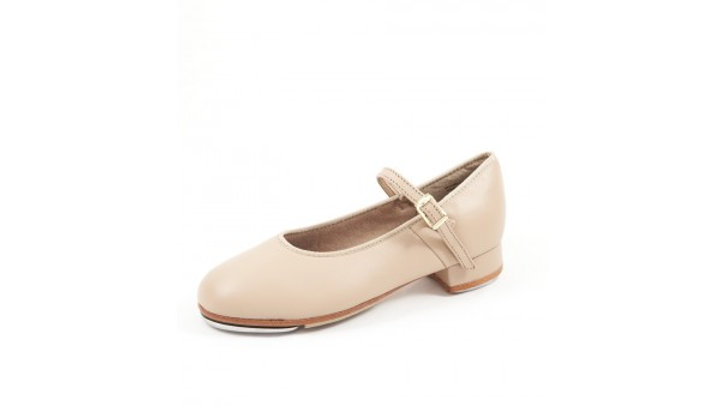# SLICKS Tap Shoes - Regular Class

`		PLEASE HAVE YOUR FOOT TRACED BY ONE OF OUR TEACHERS FOR SIZING.		Premium quality leather upper and sole with micro fibre lining	Adjustable quick release buckle	Fitted with Slick ACOUS-TECHS toe and heel plates for the ultimate in sound						US Size			 			CM							12			=			18.8cm							12.5			=			19.2cm							13			=			19.6cm							13.5			=			20cm							1			=			20.4cm							1.5			=			20.8cm							2			=			21.2cm							2.5			=			21.6cm			USSize3=22cm3.5=22.3cm4=22.7cm4.5=23.1cm5=23.5cm5.5=23.9cm6=24.3cm6.5=24.7cm7=25.1cm7.5=25.5cm8=25.9`
AU\$65.00Price
Size
Colour